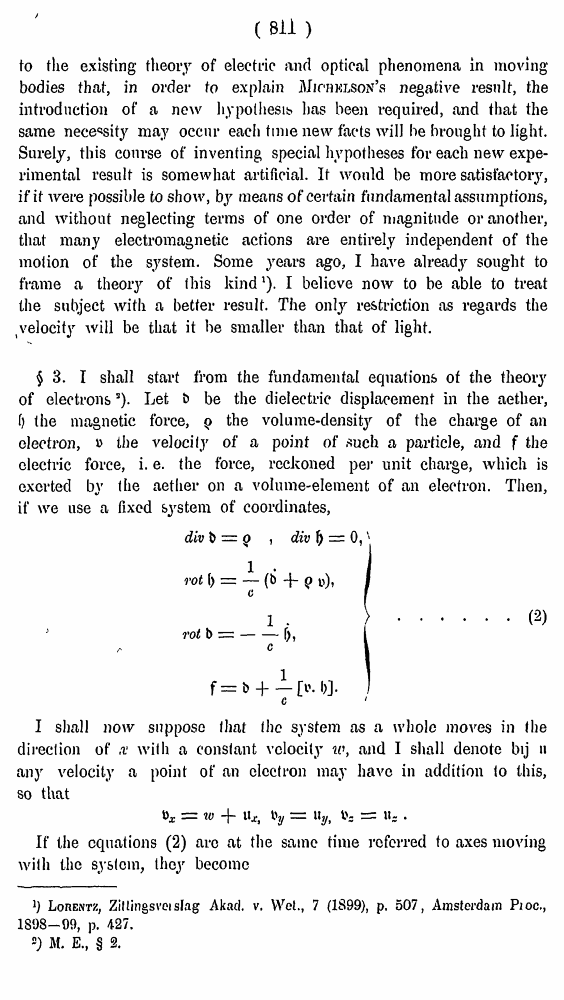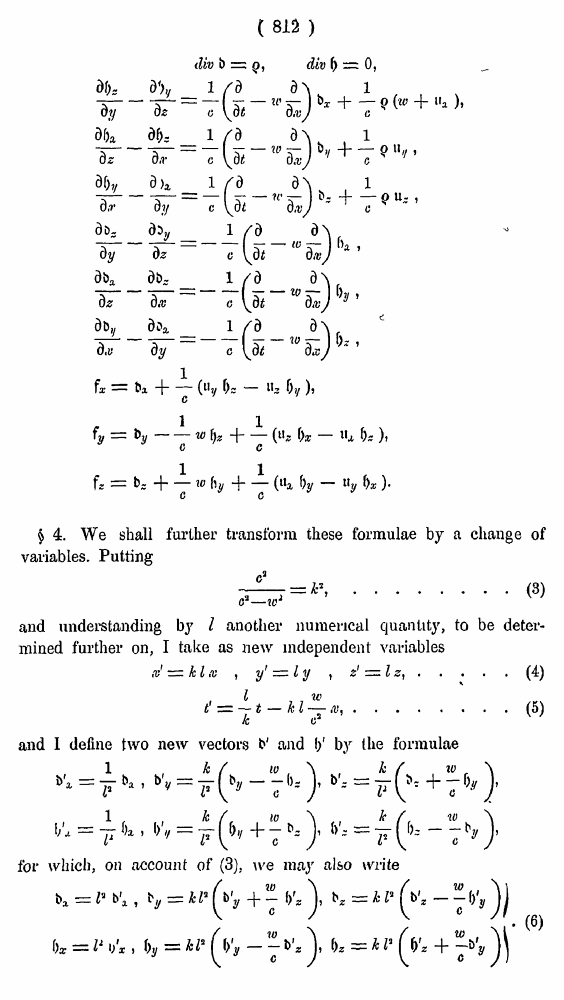# H. A. Lorentz. Electromagnetic phenomena in a system moving with any velocity smaller than that of light. // Proceedings Royal Acad., Amsterdam. Vol. VI., 1904

В начало   Другие форматы   <<<     Страница 811   >>>809  810  811 812  813  814  815  816  817  818  819  820  821  822  823  824  825  826  827  828  829  830  831
###### (sn >

to the existing theory of electric and optical phenomena in moving bodies that, in order to explain Michelson’s negative result, the introduction of a new hypothesis has been required, and that the same necessity may occur each time new facts will be brought to light. Surely, this course of inventing special hypotheses for each new experimental result is somewhat artificial. It would be more satisfactory, if if were possible to show, by means of certain fundamental assumptions, and without neglecting terms of one order of magnitude or another, that many electromagnetic actions are entirely independent of the motion of the system. Some years ago, I have already sought to frame a theory of this kind1). I believe now to be able to treat the subject with a better result. The only restriction as regards the velocity will be that it be smaller than that of light.

\$3. I shall start from the fundamental equations of the theory of electrons3). Let & be the dielectric displacement in the aether, f) the magnetic force, q the volume-density of the charge of an electron, » the velocity of a point of such a particle, and f the electric force, i. e. the force, reckoned per unit charge, which is exerted bv the aether on a volume-element of an electron. Then, if we use a fixed system of coordinates,

I shall now suppose I hat the system as a whole moves in the direction of x with a constant velocity to, and I shall denote bij h any velocity a point of an electron may have in addition to this, so that

If the equations (2) arc at the same time referred to axes moving with the system, they become

», = «> + u* i>y = %, .

]) Lorentz, Zil lings vet slag Akad. v. Wet., 7 (1899), p. 507, Amsterdam Proc., 1898-09, p. 427.

2) M. E., § 2.

( 815 )

\$ 4. We shall further transform these formulae by a change of variables. Putting

........(3)

and understanding by I another numerical quantity, to be determined further on, I take as new independent variables

.....(4)

.....(5)

and I define two new vectors b' and I)' by the formulae

tor which, on account of (3), we may also write